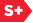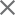### Proceedings Paper

Recovery guarantees for low complexity models (Conference Presentation)
Author(s): Samuel Vaiter; Gabriel Peyré; Jalal Fadili

Paper Abstract

This paper studies least-square regression penalized with partly smooth convex regularizers. This class of penalty functions is very large and versatile, and allows to promote solutions conforming to some notion of low-complexity. Indeed, such penalties/regularizers force the corresponding solutions to belong to a low-dimensional manifold (the so-called model) which remains stable when the penalty function undergoes small perturbations. Such a good sensitivity property is crucial to make the underlying low-complexity (manifold) model robust to small noise. In a deterministic setting, we show that a generalized "irrepresentable condition" implies stable model selection under small noise perturbations in the observations and the design matrix, when the regularization parameter is tuned proportionally to the noise level. We also prove that this condition is almost necessary for stable model recovery. We then turn to the random setting where the design matrix and the noise are random, and the number of observations grows large. We show that under our generalized "irrepresentable condition", and a proper scaling of the regularization parameter, the regularized estimator is model consistent. In plain words, with a probability tending to one as the number of measurements tends to infinity, the regularized estimator belongs to the correct low-dimensional model manifold. This work unifies and generalizes a large body of literature, where model consistency was known to hold, for instance for the Lasso, group Lasso, total variation (fused Lasso) and nuclear/trace norm regularizers. We show that under the deterministic model selection conditions, the forward-backward proximal splitting algorithm used to solve the penalized least-square regression problem, is guaranteed to identify the model manifold after a finite number of iterations. This manuscript is an excerpt from the work published in .

Paper Details

Date Published: 21 September 2017
PDF
Proc. SPIE 10394, Wavelets and Sparsity XVII, 103941V (21 September 2017); doi: 10.1117/12.2274752
Show Author Affiliations
Samuel Vaiter, Univ. De Bourgogne (France)
Ctr. National de la Recherche Scientifique (France)
Gabriel Peyré, École Normale Supérieure, DMA (France)

Published in SPIE Proceedings Vol. 10394:
Wavelets and Sparsity XVII
Yue M. Lu; Dimitri Van De Ville; Manos Papadakis, Editor(s)